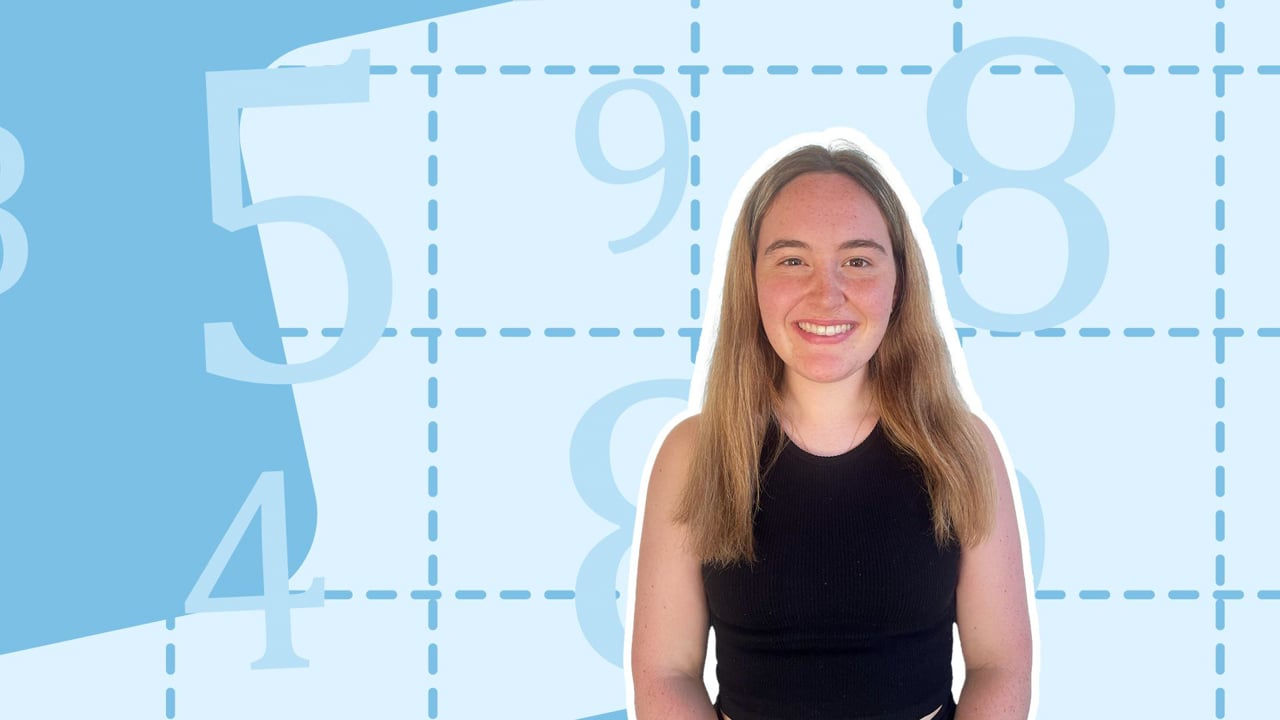Chapter OverviewMaths

Number and place value

Multiplication and division

Fractions

Measurement

Geometry - properties of shapes

Geometry - position and direction

Statistics

Maths

# Multiplying and dividing by 1 and multiplying by 00%

Summary

# Multiplying and dividing by 1 and multiplying by 0

## ​​​​​​In a nutshell

When multiplying and dividing by one or multiplying by zero, there are special rules to follow.

## Multiplying and dividing by 1

No matter what the number you want to multiply or divide by one is, the answer is always the same as the number that you started with.

##### Example 1

What is $739\times1$?

The answer is the number that you started with.

$\underline{739}$

Note: You can think of this as $739$ lots of one, or one lot of $739$.

##### Example 2

What is $652\div1$?

Again, the answer is the number that you started with.

$\underline{652}$

Note: This is like saying share $652$ into one part.

## Multiplying by 0

Multiplying by zero is just as easy - no matter what number you multiply it by, the answer is always 0.

##### Example 3

What is $43\times0$?

$\underline{0}$

Note: Unlike with one, you CANNOT divide by zero. This is an important rule in Maths.

FAQs

• Question: Can I divide by 0?

Answer: You CANNOT divide by zero. This is an important rule in maths.

• Question: What do I get when I multiply by 0?

Answer: When you multiply by zero, no matter what number you multiply it by, the answer is always 0.

• Question: What do I get when I multiply by 1?

Answer: No matter what the number you want to multiply by one, the answer is always the same as the number that you started with.

• Question: What do I get when I divide by 1?

Answer: No matter what the number you want to divide by one, the answer is always the same as the number that you started with.

Theory

Exercises